# Texas Go Math Grade 1 Lesson 4.7 Answer Key Compose 10

Refer to our Texas Go Math Grade 1 Answer Key Pdf to score good marks in the exams. Test yourself by practicing the problems from Texas Go Math Grade 1 Lesson 4.7 Answer Key Compose 10.

## Texas Go Math Grade 1 Lesson 4.6 Answer Key Compose 10

Essential Question
How can you show all of the ways to make 10?
Explanation:
The numbers must be different but the sum should be 10

Explore
Show ways to make 10. Draw to show your work.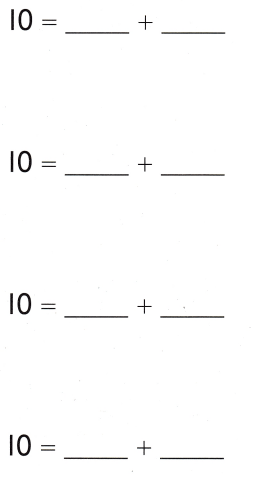Explanation:For The Teacher

• Direct children to complete the addition sentences, and draw to show ways to make 10.

Math Talk
Mathematical Processes
Explain how your drawings show make 10.
Explanation:
Joining of circles shows adding of numbers to make sum = 10

Model and Draw

He puts them in 2 boxes. How many toy cars can he put in each box?
Explanation:
5 + 5 = 10
5 toy cars can he put in each box.
to make 10Question 1.
0 + ___ = 10
0 + 9 = 10
Explanation:
The sum of two numbers is 10

Question 2.
___ + 9 = 10
1 + 9 = 10
Explanation:
The sum of two numbers is 10

Question 3.
2 + 8 = ___
2 + 8 = 10
Explanation:
The sum of two numbers is 10

Share and Show

Question 4.
3 + ___ = 10
3 + 7 = 10
Explanation:
The sum of two numbers is 10

Question 5.
___ + 6 = 10
4 + 6 = 10
Explanation:
The sum of two numbers is 10

Question 6.
5 + 5 = ___
5 + 5 = 10
Explanation:
The sum of two numbers is 10

Question 7.
6 + ___ = 10
6 + 4 = 10
Explanation:
The sum of two numbers is 10

Question 8.
___ + 3 = 10
7 + 3 = 10
Explanation:
The sum of two numbers is 10

Question 9.
8 + 2 = ___
8 + 2 = 10
Explanation:
The sum of two numbers is 10

Question 10.
9 + ___ = 10
9 + 1 = 10
Explanation:
The sum of two numbers is 10

Question 11.
___ + ___ = 10
10 + 0 = 10
Explanation:
The sum of two numbers is 10

Problem Solving

Complete the ways to make 10.

Question 12.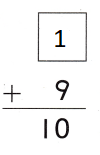Explanation:
The sum of two numbers is 10
1 + 9 = 10

Question 13.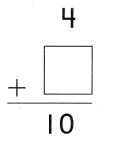Explanation:
The sum of two numbers is 10
4 + 6 = 10

Question 14.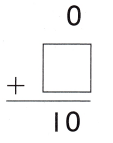Explanation:
The sum of two numbers is 10
0 + 10 = 10

Question 15.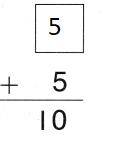Explanation:
The sum of two numbers is 10
5 + 5 = 10

Solve. Write or draw to explain.

Question 16.
Jenny has a box of 10 greeting cards. Some cards are green and 7 are purple. How many cards are green?Explanation:
7 + 3 = 10
so, 3 cards are green

Question 17.
H.O.T. Multi-Step Pablo has 2 cups on one tray and 8 cups on another tray. He wants to have the same number of cups on each tray. How many cups should be on each tray?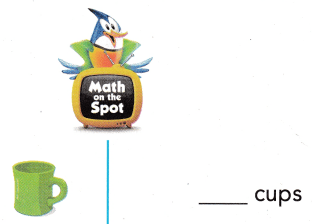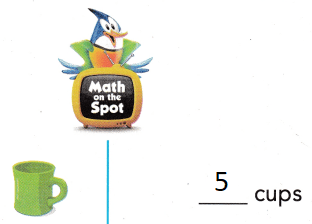Explanation:
2 + 8 = 10
10 ÷ 2 = 5
5 + 5 = 10

Question 18.
H.O.T. 10 dogs are in a show. 6 dogs jump, 2 dogs sit, and the other dogs walk. How many dogs walk?Explanation:
6 + 2 = 8
10 – 8 = 2
so, 2 dogs walk

Question 19.
Connect Chase and his sister packed 10 books in all. Which could be the number of books Chase and his sister packed?
(A) 3 books and 5 books
(B) 4 books and 4 books
(C) 9 books and 1 book
Explanation:
9 books and 1 book could be the number of books Chase and his sister packed

Question 20.
Gracie has 6 apples. How many more does she need to make 10 apples?
(A) 3
(B) 4
(C) 5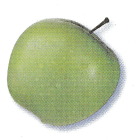Explanation:
6 + 4 = 10
4 more that she need to make 10 apples

Question 21.
Which shows a way to make 10?
(A) 1 + 0
(B) 5 + 4
(C) 7 + 3
Explanation:
7 + 3 = 10
The sum of 7 and 3 is 10

Question 22.
Texas Test Prep Which is a way to make 10?
(A) 6 + 4
(B) 9 + 0
(C) 7 + 2
Explanation:
The sum of 6 and 4 is 10
6 + 4 = 10

Take Home Activity

• Write 10 = 10 + 0. Model the problem with L pennies. Ask your child to make 10 another way. Take turns until you and your child model all of the ways to make 10.

### Texas Go Math Grade 1 Lesson 4.7 Homework and Practice Answer Key

Complete the ways to make 10.

Question 1.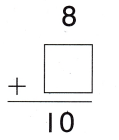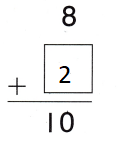Explanation:
The sum of two numbers is 10
8 + 2 = 10

Question 2.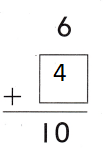Explanation:
The sum of two numbers is 10
6 + 4 = 10

Question 3.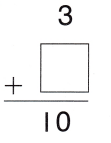Explanation:
The sum of two numbers is 10
3 + 7 = 10

Question 4.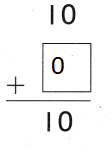Explanation:
The sum of two numbers is 10
10 + 0 = 10

Problem Solving

Write or draw to explain.

Question 5.
Will has a container of 10 model cars. Some cars are red and 5 are blue. How many cars are red?
____ red cars
5 red cars
Explanation:
10 – 5 = 5
5 + 5 = 10
so, 5 cars are red

Question 6.
10 children are in a play. 3 children sing, 3 children dance, and the others speak. How many children speak?
___ children speak
4 children speak
Explanation:
3 + 3 = 6
4 + 6 = 10
so, 4 children speak

Question 7.
Multi-Step Callie puts 7 balls in one bin and 3 balls in another bin. She wants the same number of balls in each bin. How many balls should be in each bin?
___ balls
5 balls
Explanation:
7 + 3 = 10
5 + 5 = 10
so, 5 balls will be in each bin

Texas Test Prep

Lesson Check

Question 8.(A) 2 necklaces and 8 necklaces
(B) 4 necklaces and 5 necklaces
(C) 1 necklaces and 8 necklaces
A
Explanation:
2 necklaces and 8 necklaces
2 + 8 = 10
10 could be the number of necklaces she made

Question 19.
Nick had 3 hits during the baseball game. How many more hits does he need to make 10 hits?
(A) 5
(B) 7
(C) 8
Explanation:
10 – 3 = 7
3 + 7 = 10
7 hits that he need to make 10 hits

Question 10.
Which is a way to make 10?
(A) 1 + 8
(B) 0 + 9
(C) 1 + 9
Explanation:
1 + 9 = 10
Is the correct way to make 10

Question 11.
Multi-Step Jason has 10 pennies. He puts 4 in his bank, gives 3 to his sister, and the rest to his brother. How many pennies does he give his brother?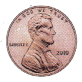(A) 2
(B) 4
(C) 3